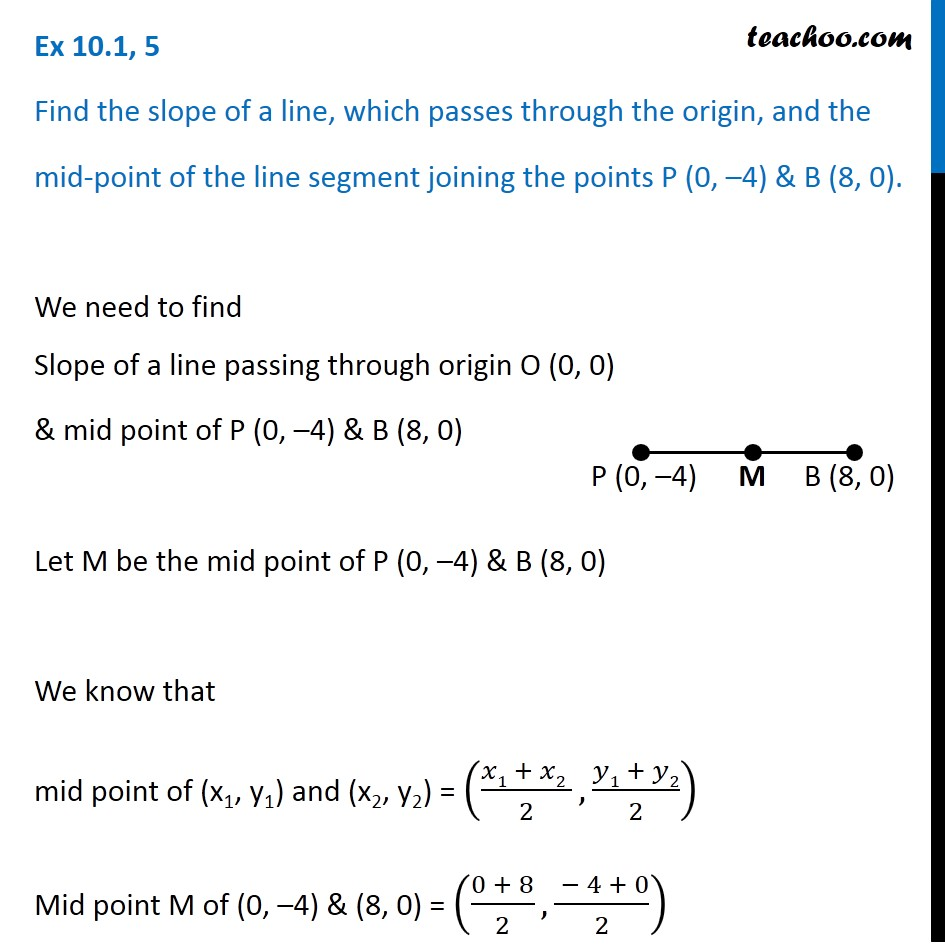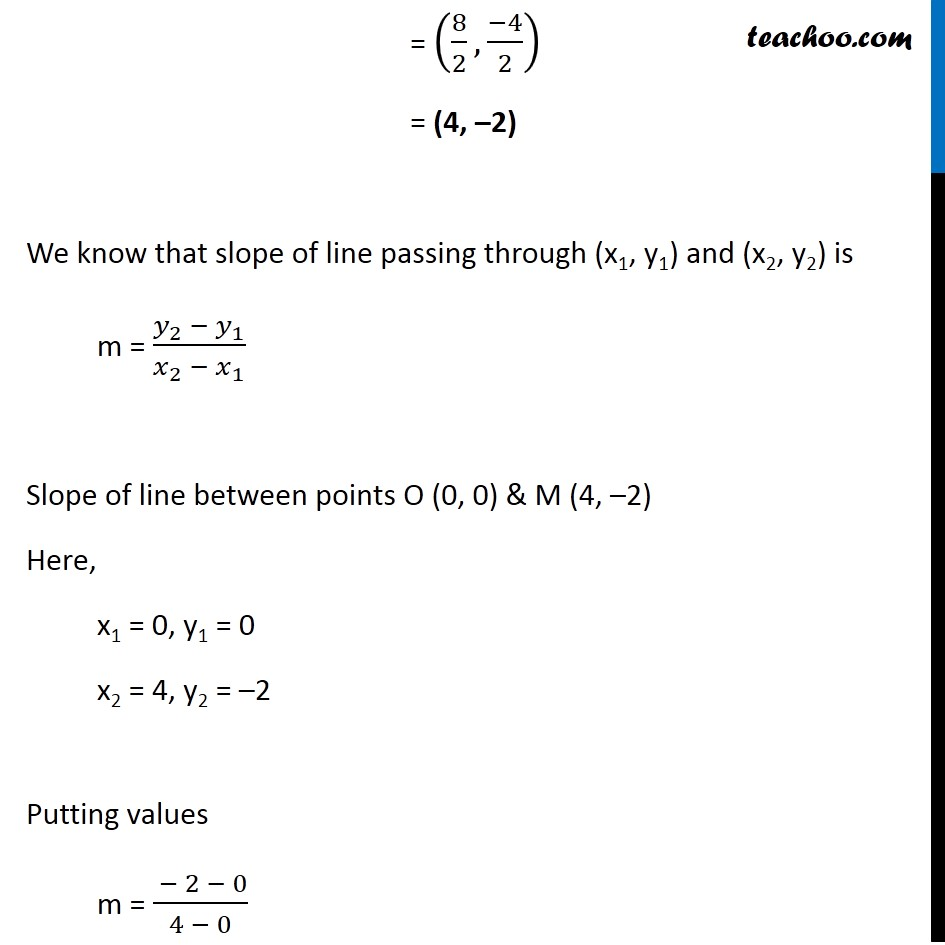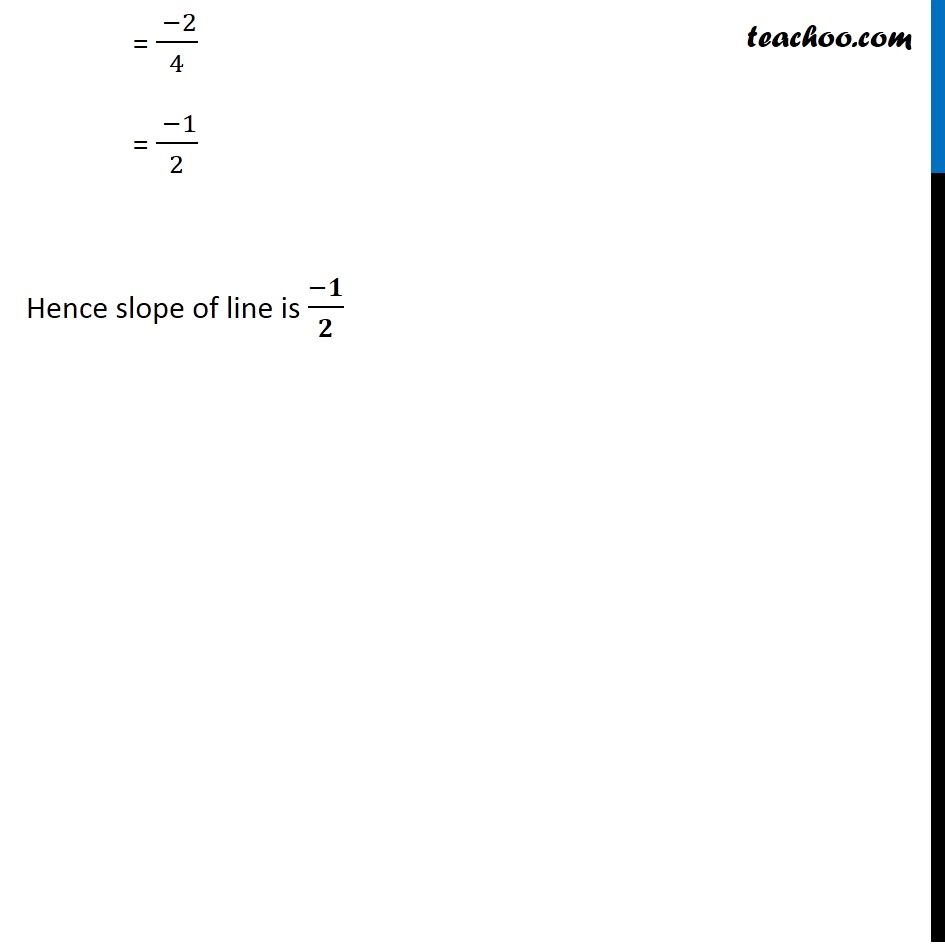Chapter 10 Class 11 Straight Lines

Class 11
Important Questions for exams Class 11Learn in your speed, with individual attention - Teachoo Maths 1-on-1 Class

### Transcript

Ex 9.1, 5 Find the slope of a line, which passes through the origin, and the mid-point of the line segment joining the points P (0, –4) & B (8, 0). We need to find Slope of a line passing through origin O (0, 0) & mid point of P (0, –4) & B (8, 0) Let M be the mid point of P (0, –4) & B (8, 0) We know that mid point of (x1, y1) and (x2, y2) = ((𝑥1 + 𝑥2 )/2,(𝑦1 + 𝑦2)/2) Mid point M of (0, –4) & (8, 0) = ((0 + 8)/2, ( − 4 + 0)/2) = (8/2, (−4)/2) = (4, –2) We know that slope of line passing through (x1, y1) and (x2, y2) is m = (𝑦_2 − 𝑦_1)/(𝑥_2 − 𝑥_1 ) Slope of line between points O (0, 0) & M (4, –2) Here, x1 = 0, y1 = 0 x2 = 4, y2 = –2 Putting values m = ( − 2 − 0)/(4 − 0) = ( −2)/4 = ( −1)/2 Hence slope of line is (−𝟏)/𝟐